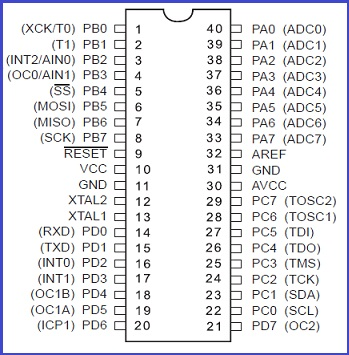# # Digitally Programming The PinThere are four sets of 8 bit programmable pin in the AVR microcontroller. They are

• PA0-7
• PB0-7
• PC0-7
• PD0-7.

These pins can be either set as input or output. Any input given or output taken is stored in register.

(figure of register contained in pin)

There are three register in AVR :

• DDR : (describe)
• PORT : (describe)
• PIN : (describe)

## # DIGITAL OUTPUT

``````#include <inttypes.h>
#include <avr/io.h>
#include <avr/interrupt.h>
#include <avr/sleep.h>

int main()
{
DDRB = 0b11111111;
while (1){
PORTB = 0b11110000;
}
return 0;
}

``````
1
2
3
4
5
6
7
8
9
10
11
12
13
14

### # Bitwise Operation:

C defines some special operations for dealing with bits. They are `&`, `|`,`^`, `<<`, `>>`,`~`.

• & (bitwise AND) : Takes two numbers as operands and does AND on every bit of two numbers. The result of AND is 1 only if both bits are 1.

• | (bitwise OR) : Takes two numbers as operands and does OR on every bit of two numbers. The result of OR is 1 any of the two bits is 1.

• ^ (bitwise XOR) : Takes two numbers as operands and does XOR on every bit of two numbers. The result of XOR is 1 if the two bits are different.

• << (left shift) : Takes two numbers, left shifts the bits of the first operand, the second operand decides the number of places to shift

• ~ (bitwise NOT) : Takes one number and inverts all bits of it

#### # Examples

Bit Shift statement in binary Resulting value in binary
0b00000001<<1 0b00000010
0b00000001<<2 0b00000100
0b00000010<<2 0b00001000
##### # Program: WAP to set 4th pin to 1 and 7th pin 0 :
``````#include <inttypes.h>
#include <avr/io.h>
#include <util/delay.h>

int main()
{

DDRA = 0b11111111;

while (1)
{
PORTA =0b11110000;
_delay_ms(1000);
//left shift operator
//00000001 << 3 = 00001000
//setting 1 to 4th pin
//OR Operator
PORTA |=1 << 3;
_delay_ms(1000);
//NOT operator
//Setting 0 to 7th pin
//AND OPERATOR
PORTA &= ~(1 << 6);
_delay_ms(1000);
//Combining Multiple Operator
PORTA |= (1 << 0)| (1 << 1) ;
_delay_ms(1000);

}
return 0;
}
``````
1
2
3
4
5
6
7
8
9
10
11
12
13
14
15
16
17
18
19
20
21
22
23
24
25
26
27
28
29
30
31
32

The people who created WinAVR also created a standard way to bit value manipulations.

``````#DEFINE _BV(bit) ( 1 << bit )
``````
1

Like #DEFINE _BV(3) means 1<<3 which is 0x08

``````PORTX = _BV(bit) | _BV(bit)
``````
1

Like PORTA = _BV(0) | _BV(7) means 1<<0 OR 1<<7 = 10000001

### # Updating single pin of the port value at a time :

``````PORTA = PORTA | _BV(3)
``````
1

It is equivalent to

``````PORTA |= _BV(3)
``````
1

Let PORTA = 0b11110000 and _BV(3) = 0b00001000 OR-ing these two makes 0b11111000 which results in updating the required port value.

### # Turning the bits off:

``````PORTA &= ~_BV(0)
``````
1

Let PORTA = 0b01010101 , then _BV(0) = 0b00000001, AND-ing the invert of _BV(0), 0b11111110, and PORTA makes 0b01010100 which results in turning the specific bit off.

### # For setting up a bit and clearing a bit, macros are defined:

``````#define setbit(port,bit) (port) |= (1 << bit)
#define clearbit(port,bit) (port) &= ~(1 << bit)

setbit(PORTA, PA0)
clearbit(PORTA, PA7)
``````
1
2
3
4
5

## # DIGITAL INPUT

Tips

“You can not detect high input from the switch without using external circuit “ HINT : Switch does not ground the the circuit when it’s off , the pin remains high even if the switch is off .

### # HIGH INPUT DETECTION (only from sensor) :

``````#include <inttypes.h>
#include <avr/io.h>
#include <avr/interrupt.h>
#include <avr/sleep.h>

int main()
{
DDRA = 0b00000000;
DDRB = 0xFF;

while (1){

if( (PINA & (1<<0)) == 1){
PORTB = 0xFF;
}
else{
PORTB = 0x00;
}
}
return 0;
}

``````
1
2
3
4
5
6
7
8
9
10
11
12
13
14
15
16
17
18
19
20
21
22

### # LOW INPUT DETECTION (for both sensor and switch) :

Let us see an input program:

``````#include <inttypes.h>
#include <avr/io.h>
#include <avr/interrupt.h>
#include <avr/sleep.h>

int main()
{
DDRA = 0b00000000;
//pull-up
PORTA = 0b11111111;
DDRB = 0xFF;

while (1){

//Masking the other pin to determine zero .
if( (PINA & (1<<0)) == 0){
PORTB = 0xFF;
}
else{
PORTB = 0x00;
}
}
return 0;
}
``````
1
2
3
4
5
6
7
8
9
10
11
12
13
14
15
16
17
18
19
20
21
22
23
24

### # STEPS

1. Make the pin opposite of the desired detection value:
• For high detection nothing to be done
• For Low just use PORTX = 0XFF (PULL UP CONCEPT)
2. Check the condition : if `((PINX & (1<<pin_number)) == detection value (1 or 0))` , and operator of 1 with anything yields the other operating values